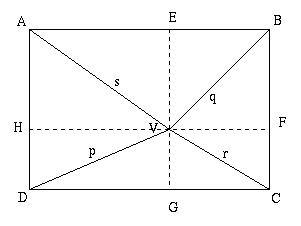#### You may also like### Some(?) of the Parts

A circle touches the lines OA, OB and AB where OA and OB are perpendicular. Show that the diameter of the circle is equal to the perimeter of the triangle### Baby Circle

A small circle fits between two touching circles so that all three circles touch each other and have a common tangent? What is the exact radius of the smallest circle?### Logosquares

Ten squares form regular rings either with adjacent or opposite vertices touching. Calculate the inner and outer radii of the rings that surround the squares.

# Rectangular Pyramids

##### Age 14 to 18 Challenge Level:

A proof of this result came from students from the Key Stage 3 Maths Club at Strabane Grammar School, Northern Ireland and the following one from Joel, ACS (Barker) Singapore:(a) In the diagram given (in 2 dimensions) p 2 + q 2 = r 2 + s 2

Proof

Draw 2 lines through V parallel to the edges of the rectangle, dividing the figure into four pairs of right-angled triangles. Label the point on AB as E, on BC as F, on CD as G and on DA as H. By Pythagoras theorem:

 p 2 = DG 2 +DH 2 q 2 = BE 2 + BF 2 r 2 = CF 2 + CG 2 s 2 = AE 2 + AH 2

Since DG=AE, CG=BE, AH=BF and CF=DH,

p 2 + q 2 = AE 2 + CF 2 + CG 2 + AH 2 = r 2 + s 2

(b) If the diagram represents a pyramid on a rectangular base where p, q, r and s are the lengths of the sloping edges then the result p 2 + q 2 = r 2 + s 2 still holds true.

Proof

Let V 1 be the foot of the perpendicular from V to the base ABCD of the pyramid and let h be the height of the pyramid so that VV 1 = h and let V 1 A = s 1 , V 1 B = q 1 , V 1 C = r 1 , and V 1 D= p 1 .

By Pythagoras theorem we have: p 1 2 + h 2 = p 2 , q 1 2 + h 2 = q 2 , r 1 2 + h 2 = r 2 and s 1 2 + h 2 = s 2 .

Using the result already proved in 2dimensions, that is

p 1 2 + q 1 2 = r 1 2 + s 1 2 ,

we get p 1 2 + q 1 2 + 2h 2 = r 1 2 + s 1 2 + 2h 2

so p 2 + q 2 = r 2 + s 2 .